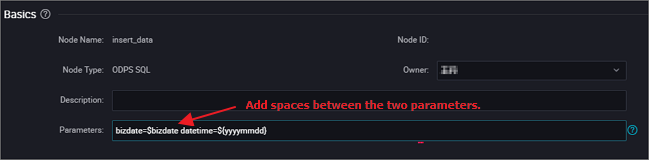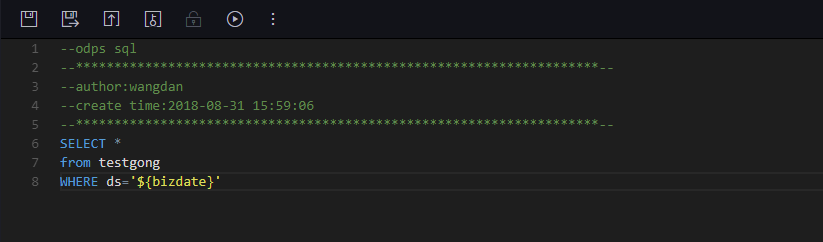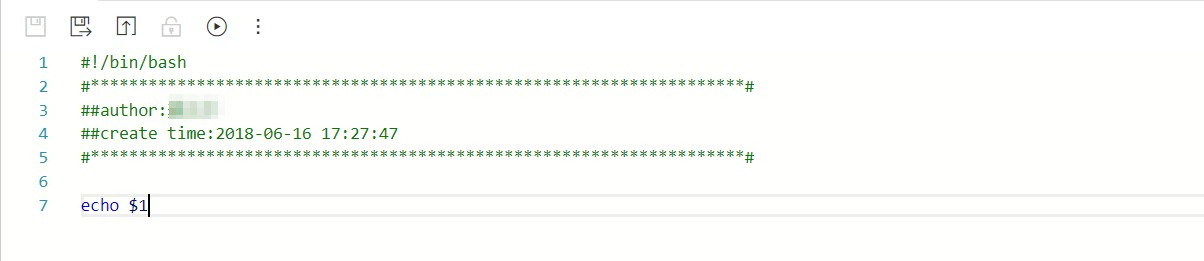To ensure that tasks can dynamically adapt to environment changes when running automatically at the scheduled time, DataWorks provides the parameter configuration feature. Pay special attention to the following issues before configuring parameters.
• No space can be added on either side of the equation mark "=" of a parameter. Correct: bizdate=\$bizdate• Multiple parameters (if any) must be separated by spaces.## System parameters

DataWorks provides two system parameters, which are defined as follows:
• \${bdp.system.cyctime}: It is defined as the scheduled run time of an instance. Default format: yyyymmddhh24miss.
• \${bdp.system.bizdate}: It is defined as the business date on which an instance is calculated. Default business data is one day before the running date, which is displayed in default format: yyyymmdd.

According to the definitions, the formula for calculating the runtime and business date is as follows: `Runtime = Business date - 1`.

To use the system parameters, directly reference '\${bizdate}' in the code without setting system parameters in the editing box, and the system will automatically replace the reference fields of system parameters in the code.
Note The scheduling attribute of a periodic task is configured with a scheduled runtime. Therefore, you can backtrack the business date based on the scheduled runtime of an instance and retrieve the values of system parameters for the instance.

## Example

Set an ODPS_SQL task that runs every hour between 00:00 and 23:59 every day. To use system parameters in the code, perform the following statement.
``````insert overwrite table tb1 partition(ds ='20180606') select
c1,c2,c3
from (
select * from tb2
where ds ='\${bizdate}');``````

## Configure scheduling parameters for a non-Shell node

Note The name of a variable in the SQL code can contain only a-z, A-Z, numbers, and underlines. If the variable name is "date", the value "\$bizdate" is automatically assigned to this variable, and you do not need to assign the value in the scheduling parameter configuration. Even if another value is assigned, this value is not used in the code because the value "\$bizdate" is automatically assigned in the code by default.

For a non-Shell node, you need to first add \${variable name} (indicating that the function is referenced) in the code, then input a specific value to assign the value to the scheduling parameter.

For example, for an ODPS SQL node, add \${variable name} in the code, and then configure the parameter item "variable name=built-in scheduling parameter" for the node.

For a parameter referenced in the code, you must add the parsed value during scheduling.## Configure scheduling parameters for a Shell node

The parameter configuration procedure of a Shell node is similar to that of a non-Shell node except that rules are different. For a Shell node, variable names cannot be customized and must be named '\$1,\$2,\$3...'.

For example, for a Shell node, the Shell syntax declaration in the code is: \$1, and the node parameter configuration in scheduling is: \$xxx (built-in scheduling parameter). That is, the value of \$xxx is used to replace \$1 in the code.

For a parameter referenced in the code, you must add the parsed value during scheduling.Note For a Shell node, when the number of parameters reaches 10, \${10} should be used to declare the variable.

## The variable value is a fixed value

Take an SQL node for example. For \${variable name} in the code, configure the parameter item "variable name="fixed value"" for the node.

Code: select xxxxxx type=’\${type}’

Value assigned to the scheduling variable: type="aaa"

During scheduling, the variable in the code is replaced by type='aaa'.

## The variable value is a built-in scheduling parameter

Take an SQL node for example. For \${variable name} in the code, configure the parameter item variable name=scheduling parameter for the node.

Code: select xxxxxx dt=\${datetime}

alue assigned to the scheduling variable: datetime=\$bizdate

During scheduling, if today is July 22, 2017, the variable in the code is replaced by dt=20170721.

## Built-in scheduling parameter list

\$bizdate: business date in the format of yyyymmdd NOTE: This parameter is widely used, and is the date of the previous day by default during routine scheduling.

For example: In the code of the ODPS SQL node, pt=\${datetime}. In the parameter configuration of the node, datetime=\$bizdate. Today is July 22, 2017. When the node is executed today, \$bizdate is replaced by pt=20170721.

For example, In the code of the ODPS SQL node, pt=\${datetime}. In the parameter configuration of the node, datetime=\$gmtdate. Today is July 22, 2017. When the node is executed today, \$gmtdate is replaced by pt=20170722.

For example, In the code of the ODPS SQL node, pt=\${datetime}. In the parameter configuration of the node, datetime=\$bizdate. Today is July 1, 2017. When the node is executed today, \$bizdate is replaced by pt=20130630.

For example, In the code of the ODPS SQL node, pt=\${datetime}. In the parameter configuration of the node, datetime=\$gmtdate. Today is July 1, 2017. When the node is executed today, \$gmtdate is replaced by pt=20170701.

\$cyctime: scheduled time of the task. If no scheduled time is configured for a daily task, cyctime is 00:00 of the current day. The time is accurate to hour, minute, and second, and is generally used for a hour-level or minute-level scheduling task. Example: cyctime=\$cyctime.\$jobid: ID of the workflow to which a task belongs. Example: jobid=\$jobid.

\$nodeid: ID of a node. Example: nodeid=\$nodeid.

\$taskid: ID of a task, that is, ID of a node instance. Example: taskid=\$taskid.

\$bizmonth: business month in the format of yyyymm.
• If the month of a business date is equal to the current month, \$bizmonth = Month of the business date - 1; otherwise, \$bizmonth = Month of the business date.
• For example: In the code of the ODPS SQL node, pt=\${datetime}. In the parameter configuration of the node, datetime=\$bizmonth. Today is July 22, 2017. When the node is executed today, \$bizmonth is replaced by pt=201706.

\$gmtdate: current date in the format of yyyymmdd. The value of this parameter is the current date by default. During data population, gmtdate that is input is the business date plus 1.

Custom parameter \${…} Parameter description:
• Time format customized based on \$bizdate, where yyyy indicates the 4-digit year, yy indicates the 2-digit month, mm indicates the month, and dd indicates the day. The parameter can be combined as expected, for example, \${yyyy}, \${yyyymm}, \${yyyymmdd}, \${yyyy-mm-dd}.
• \$bizdate is accurate to year, month, and day. Therefore, the custom parameter \${……} can only represent the year, month, or day.
• Methods for obtaining the period plus or minus certain duration:

Next N years: \${yyyy+N}

Previous N years: \${yyyy-N}

Next N months: \${yyyymm+N}

Previous N months: \${yyyymm-N}

Next N weeks: \${yyyymmdd+7*N}

Previous N weeks: \${yyyymmdd-7*N}

Next N days: \${yyyymmdd+N}

Previous N days: \${yyyymmdd-N}

\${yyyymmdd}: business date in the format of yyyymmdd. The value is consistent with that of \$bizdate.
• Note: The value is consistent with that of \$bizdate. This parameter is widely used, and is the date of the previous day by default during routine scheduling.  The format of this parameter can be customized, for example, the format of \${yyyy-mm-dd} is yyyy-mm-dd.
• For example: In the code of the ODPS SQL node, pt=\${datetime}. In the parameter configuration of the node, datetime=\${yyyymmdd}. Today is July 22, 2013. When the node is executed today, \${yyyymmdd} is replaced by pt=20130721.

\${yyyymmdd-/+N}: yyyymmdd plus or minus N days

\${yyyymm-/+N}: yyyymm plus or minus N month

\${yyyy-/+N}: year (yyyy) plus or minus N years

\${yy-/+N}: year (yy) plus or minus N years

NOTE: yyyymmdd indicates the business date and supports any separator, such as yyyy-mm-dd. The preceding parameters are derived from the year, month, and day of the business date.

Example:

• In the code of the ODPS SQL node, pt=\${datetime}. In the parameter configuration of the node, datetime=\${yyyy-mm-dd}. Today is July 22, 2018. When the node is executed today, \${yyyy-mm-dd} is replaced by pt=2018-07-21.
• In the code of the ODPS SQL node, pt=\${datetime}. In the parameter configuration of the node, datetime=\${yyyymmdd-2}. Today is July 22, 2018. When the node is executed today, \${yyyymmdd-2} is replaced by pt=20180719.
• In the code of the ODPS SQL node, pt=\${datetime}. In the parameter configuration of the node, datetime=\${yyyymm-2}. Today is July 22, 2018. When the node is executed today, \${yyyymm-2} is replaced by pt=201805.
• In the code of the ODPS SQL node, pt=\${datetime}. In the parameter configuration of the node, datetime=\${yyyy-2}. Today is July 22, 2018. When the node is executed today, \${yyyy-2} is replaced by pt=2018.

In the ODPS SQL node configuration, multiple parameters are assigned values, for example, startdatetime=\$bizdate enddatetime=\${yyyymmdd+1} starttime=\${yyyy-mm-dd} endtime=\${yyyy-mm-dd+1}.

Example: (Assume \$cyctime=20140515103000)
• \$[yyyy] = 2014, \$[yy] = 14, \$[mm] = 05, \$[dd] = 15, \$[yyyy-mm-dd] = 2014-05-15, \$[hh24:mi:ss] = 10:30:00, \$[yyyy-mm-dd hh24:mi:ss] = 2014-05-1510:30:00
• \$[hh24:mi:ss - 1/24] = 09:30:00
• \$[yyyy-mm-dd hh24:mi:ss -1/24/60] = 2014-05-1510:29:00
• \$[yyyy-mm-dd hh24:mi:ss -1/24] = 2014-05-1509:30:00
• \$[add_months(yyyymmdd,-1)] = 2014-04-15
• \$[add_months(yyyymmdd,-12*1)] = 2013-05-15
• \$[hh24] =10
• \$[mi] =30

Method for testing the parameter \$cyctime:

After the instance runs, right-click the node to check the node attribute. Check whether the scheduled time is the time at which the instance runs periodically.

Result after the parameter value is replaced by the scheduled time minus one hour.